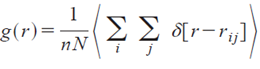# RDF formula in Lammps

Dear Lammps users,

I could not find a formula for calculating RDF in Lammps. Would like to know if it is the same as the bellow formulaN: total number of ion pairs

n: number of density

rij: distance between atoms i and j

I asked because I am simulating a paper, they used the above formula for calculating their RDF. I also calculated RDF for the crystal by Lammps, the peak positions are exactly the same as the paper but their intensity is very different even their relative heights. Would like to know if I need to do some normalization?

Regards,

Sara

Dear Lammps users,

I could not find a formula for calculating RDF in Lammps. Would like to
know if it is the same as the bellow formula

N: total number of ion pairs

n: number of density

rij: distance between atoms i and j

​yes, it pretty much is. there only is an additional correct for finite
size effect, so that g(r) for r -> r_c becomes exactly 1.0 in a dense,
homogeneous, and liquid mono-atomic system.​

I asked because I am simulating a paper, they used the above formula for
calculating their RDF. I also calculated RDF for the crystal by Lammps, the
peak positions are exactly the same as the paper but their intensity is
very different even their relative heights. Would like to know if I need to
do some normalization?

​no, but the exact results also depend on the details of what kind of pairs
are considered. this is particularly crucial for any system with bonds.

axel.Hi Axel,

I considered the pairs of atoms exactly the same as the paper. Would you please see the attached file. I appreciate if you have more comments for me.

Kind regards,
SaraComparing RDFs.docx (170 KB)

Hi Axel,

I considered the pairs of atoms exactly the same as the paper. Would you
please see the attached file. I appreciate if you have more comments for
me.

not really. i suggest you first use compute rdf with a simpler system to
confirm that you are using it correctly.
it has been benchmarked against multiple different RDF implementations and
come out to work correctly in all cases.​ this includes the RDF code
implementations in VMD on GPU and CPU, the latter of which i wrote myself
based on a code i wrote and extensively tested during my own PhD studies
almost 20 years ago. the code in VMD has seen some close scrutiny from a
lot of people and over the years.

axel.Hi all,

The system you are studying is solid, so results should be dependent on size of bin, i.e. delta_r is used to numerically compute g®. So, I think that first you should test with different number of bins!

Best,That’s a good point, about the number of bins. Hopefully the authors specified the number of bins they used (wistful thinking probably).